# QPSK Digital Modulation Simulation Using MATLAB: Quadrature Phase Shift Keying

Quadrature Phase Shift Keying (QPSK) is one of  the type of phase shift keying. Unlike BPSK which send one bit information at an instance, QPSK is a modulation which sends two bits of digital information a time (without the use of another carrier frequency). They both are by the way DSBCS modulation scheme.

In a sense, QPSK is an expanded version from binary PSK where in a symbol consists of two bits and two orthonormal basis functions are used. A group of two bits is often called a 'dibit'. So, four dibits are possible. Each symbol carries same energy.

The above was just in introduction about what QPSK is, search over the internet to get more stuffs about it. You will get number of learning resources which explains you the A-Z of the theoretical aspect of it.

The process which we have followed while implementing on MATLAB is quite conventional.
First: get the bit stream.
Then Divide the bit stream in 2 bit streams: even & odd.
Then apply the BPSK techniques on these 2 bit streams separately.
Add the modulated waves to get the QPSK.

Another way to implement it in MATLAB or any other simulation software would be, to introduce a phase shift of  90 degree, on each pair of bit change. For more information just search Maxim Integrated QPSK tutorial, here you will get all the relevant equation information.

MATLAB Code For QPSK :

clc;
clear all;
close all;
cvx=input('Enter Length of Random Bit Sequence:');
d=round(rand(1,cvx))
l=cvx;
x=0:0.01:l*2*pi;
cc=cos(x);
cs=cos(x+pi/2);
k=length(cc);
k1=k/l;

for i=1:l
if(d(i)==0)
d(i)=-1;
i=i+1;
end
end

i=1;
j=1;
% To segrigate odd bit streams and even bit streams
while (i<l) && (j<l)
%half strem with double symbol duration
dd1(j)=d(i);
dd1(j+1)=d(i);
dd2(j)=d(i+1);
dd2(j+1)=d(i+1);
j=j+2;
i=i+2;
end
% to make bit streams cycle equivalent to sinusoidal waveformt=1;
for i=1:l
for j=1:k1
%k1 sample with 1 sine period
dd(t)=d(i);
d1(t)=dd1(i);
d2(t)=dd2(i);
t=t+1;
j=j+1;
end
i=i+1;
end

subplot(6,1,1);
stairs(dd);
axis([0 t -2 2]);
title('Imput Bit Stream');
subplot(6,1,2);
stairs(d1);
axis([0 t -2 2]);
title('Odd Bit Stream');
subplot(6,1,3);
stairs(d2);
axis([0 t -2 2]);
title('Even Bit Stream');

len=length(d1);
if(k<len)
len=k;
end

for i=1:len
qcc(i)=cc(i)*d1(i);
% odd streams multiplied with I waveform
qcs(i)=cs(i)*d2(i);
% even streams multiplied with Q waveform
i=i+1;
end

subplot(6,1,4);
plot(qcc);
axis([0 len -2 2]);
title('Modulated Wave of I-Component');
subplot(6,1,5);
plot(qcs);
axis([0 len -2 2]);
title('Modulated Wave of Q-Component');

qp=qcc+qcs;
subplot(6,1,6);
plot(qp);
axis([0 len -2 2]);
title('QPSK WAVEFORM');
figure, scatter(dd1,dd2,40,'*r');
title('Constellation Diagram of QPSK');

Results: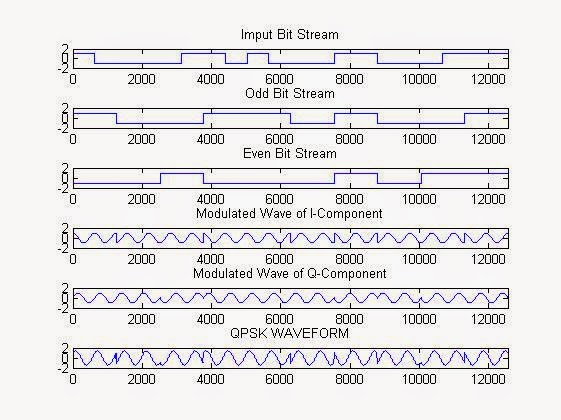QPSK MATLAB Simulation Result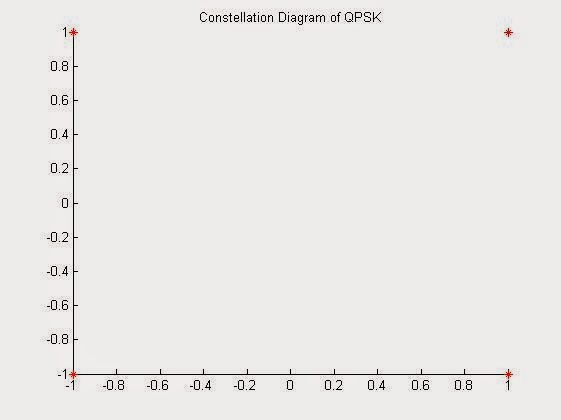QPSK Constellation Plot

# Sound Record, Save & Playback in MATLAB

Yes Matlab is also capable to handle the devices that are connected to the computer system ports. It can gather data from these devices.
In this example we will gather sound data from the microphone connected to your system. The code is optimized for the system which is having more than one audio input device connected to them. In that case, although from a single instance of the code run you will be able to collect data only from one of the device (will be selected though the device id). But if run multiple instance of MATLAB with the same code you will be able to record simultaneously from different input devices.

The following program will require you to select the device id, set the duration of the recoding, & the bit depth of recording during runtime.
The recording is happening in the sampling rate of 48kHz & dual channel, i.e., stereo. The audio data will be stored by the name 'xyz.wav'.

NOTE: you can select different sampling rate, that is supported by your recording device (or sound card) just see the microphone properties in "Control Panel".

MATLAB code for  Sound Record, Save & Playback:

clc;
clear all;
close all;
t=audiodevinfo;
%getting audio device status connected with the computer system
n=size(t.input,2); %getting especially the input device status
disp('Details Of Audio Recording device Connected:');
for i=0:n-1
disp('Device Name:')

disp(t.input(1,i+1).Name) %display the audio input device name
disp('Device id:')
disp(i)
%diaplay the device id
end
d=input('Enter your Device ID from the above Device List to Start Recording:');
%getting the device id for recording
t=input('Enter the Duration of Recording(s):');
%getting the duration of recording
b=input('Enter Bit Depth of Recording(e.g. 8bit):'); %getting the bit depth of recording
disp('Recording at 48kHz Sampling frequency');
aud=audiorecorder(48000,b,2,d);
disp('Recording....')
recordblocking(aud,t);
myRecording = getaudiodata(aud);
%start audio recoding
plot(myRecording); %plot the recorded data
audiowrite('xyz.wav',myRecording, 48000); %write the recorded audio to a file
disp('Saving the Audio File as xyz.wav in the working directory...!');
disp('Playing....');
play(aud);
%play the recorded file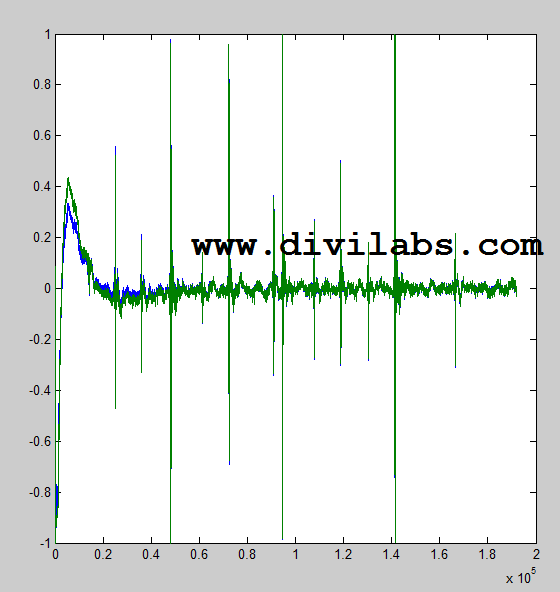Sound Record Plot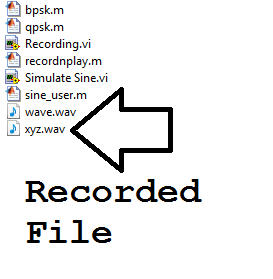Recorded File: As seen on Explorer for the current working directory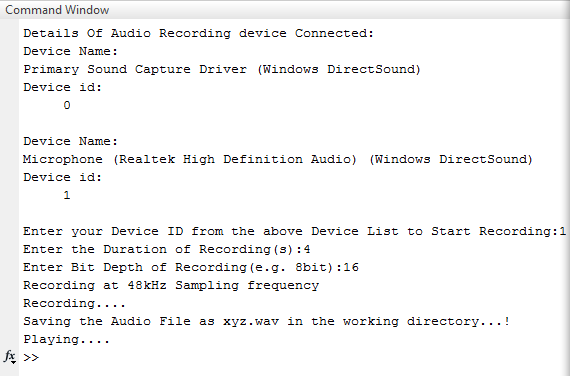Audio Recorder in Action